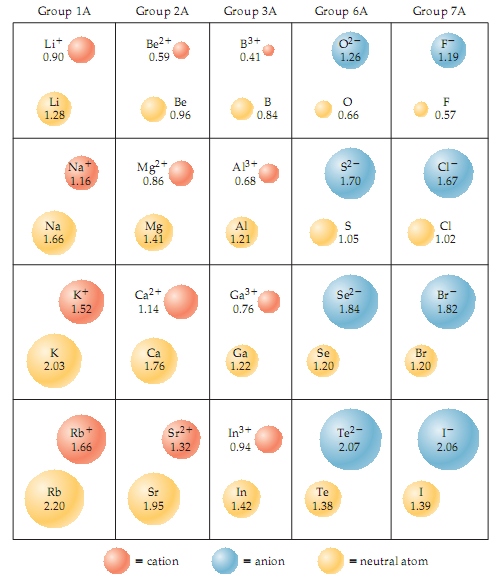# Problem: Consider the ionic compounds KF, NaCl, NaBr, and LiCl.Use ionic radii to estimate the cation-anion distance for each compound.

###### FREE Expert Solution

We’re asked to estimate the cation-anion distance for each compound using the given ionic radii

Recall that we can estimate the cation-anion distance by getting the sum of the ionic radius of the cation and anion in an ionic compound.

The ionic radius of each ion in each given compound can be found on the given table.

98% (78 ratings)###### Problem Details

Consider the ionic compounds KF, NaCl, NaBr, and LiCl.Use ionic radii to estimate the cation-anion distance for each compound.